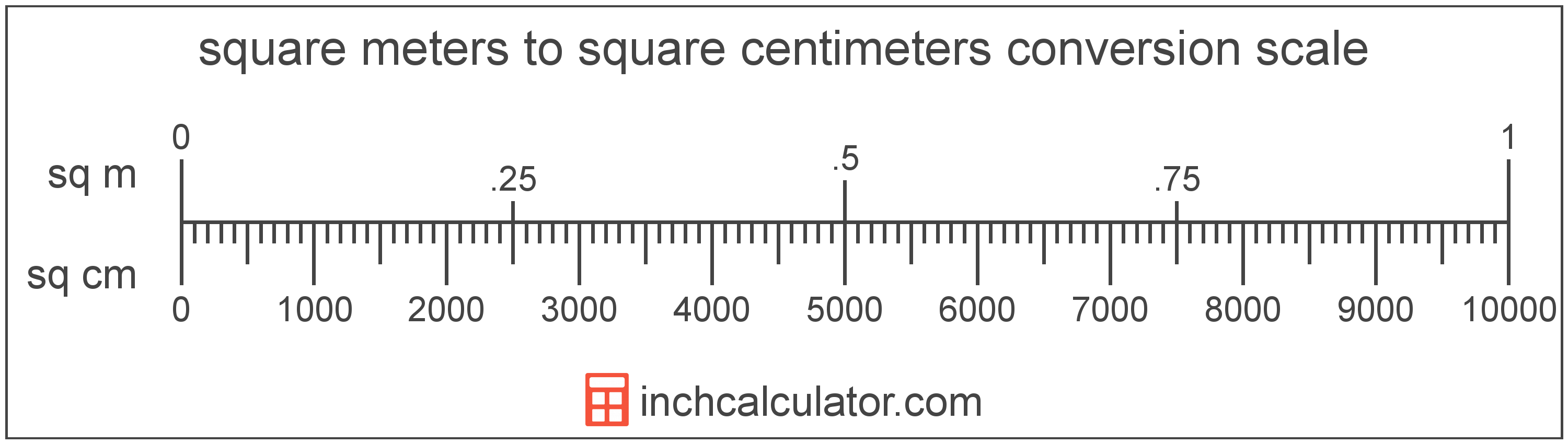# Square Meters to Square Centimeters Conversion

Enter the area in square meters below to get the value converted to square centimeters.

(find square meters)
Results in Square Centimeters:1 sq m = 10,000 sq cm

## How to Convert Square Meters to Square CentimetersTo convert a square meter measurement to a square centimeter measurement, multiply the area by the conversion ratio.

Since one square meter is equal to 10,000 square centimeters, you can use this simple formula to convert:

square centimeters = square meters × 10,000

The area in square centimeters is equal to the square meters multiplied by 10,000.

For example, here's how to convert 5 square meters to square centimeters using the formula above.
5 sq m = (5 × 10,000) = 50,000 sq cm

### How Many Square Centimeters are in a Square Meter?

There are 10,000 square centimeters in a square meter, which is why we use this value in the formula above.

1 sq m = 10,000 sq cm

## Square Meters

One square meter is equal to the area of a square with sides that are 1 meter in length.

The square meter, or square metre, is the SI derived unit for area in the metric system. A square meter is sometimes also referred to as a square m. Square meters can be abbreviated as sq m, and are also sometimes abbreviated as . For example, 1 square meter can be written as 1 sq m or 1 m².

Try our square meters calculator to calculate the area of a space.

## Square Centimeters

One square centimeter is equal to the area of a square with 1 centimeter long sides.

The square centimeter, or square centimetre, is a multiple of the square meter, which is the SI derived unit for area. In the metric system, "centi" is the prefix for 10-2. A square centimeter is sometimes also referred to as a square cm. Square centimeters can be abbreviated as sq cm, and are also sometimes abbreviated as cm². For example, 1 square centimeter can be written as 1 sq cm or 1 cm².

## Square Meter to Square Centimeter Conversion Table

Square meter measurements converted to square centimeters
Square Meters Square Centimeters
0.0001 sq m 1 sq cm
0.0002 sq m 2 sq cm
0.0003 sq m 3 sq cm
0.0004 sq m 4 sq cm
0.0005 sq m 5 sq cm
0.0006 sq m 6 sq cm
0.0007 sq m 7 sq cm
0.0008 sq m 8 sq cm
0.0009 sq m 9 sq cm
0.001 sq m 10 sq cm
0.002 sq m 20 sq cm
0.003 sq m 30 sq cm
0.004 sq m 40 sq cm
0.005 sq m 50 sq cm
0.006 sq m 60 sq cm
0.007 sq m 70 sq cm
0.008 sq m 80 sq cm
0.009 sq m 90 sq cm
0.01 sq m 100 sq cm
0.02 sq m 200 sq cm
0.03 sq m 300 sq cm
0.04 sq m 400 sq cm
0.05 sq m 500 sq cm
0.06 sq m 600 sq cm
0.07 sq m 700 sq cm
0.08 sq m 800 sq cm
0.09 sq m 900 sq cm
0.1 sq m 1,000 sq cm
0.2 sq m 2,000 sq cm
0.3 sq m 3,000 sq cm
0.4 sq m 4,000 sq cm
0.5 sq m 5,000 sq cm
0.6 sq m 6,000 sq cm
0.7 sq m 7,000 sq cm
0.8 sq m 8,000 sq cm
0.9 sq m 9,000 sq cm
1 sq m 10,000 sq cm

## References

1. Collins Dictionary, Definition of 'square meter', https://www.collinsdictionary.com/us/dictionary/english/square-meter